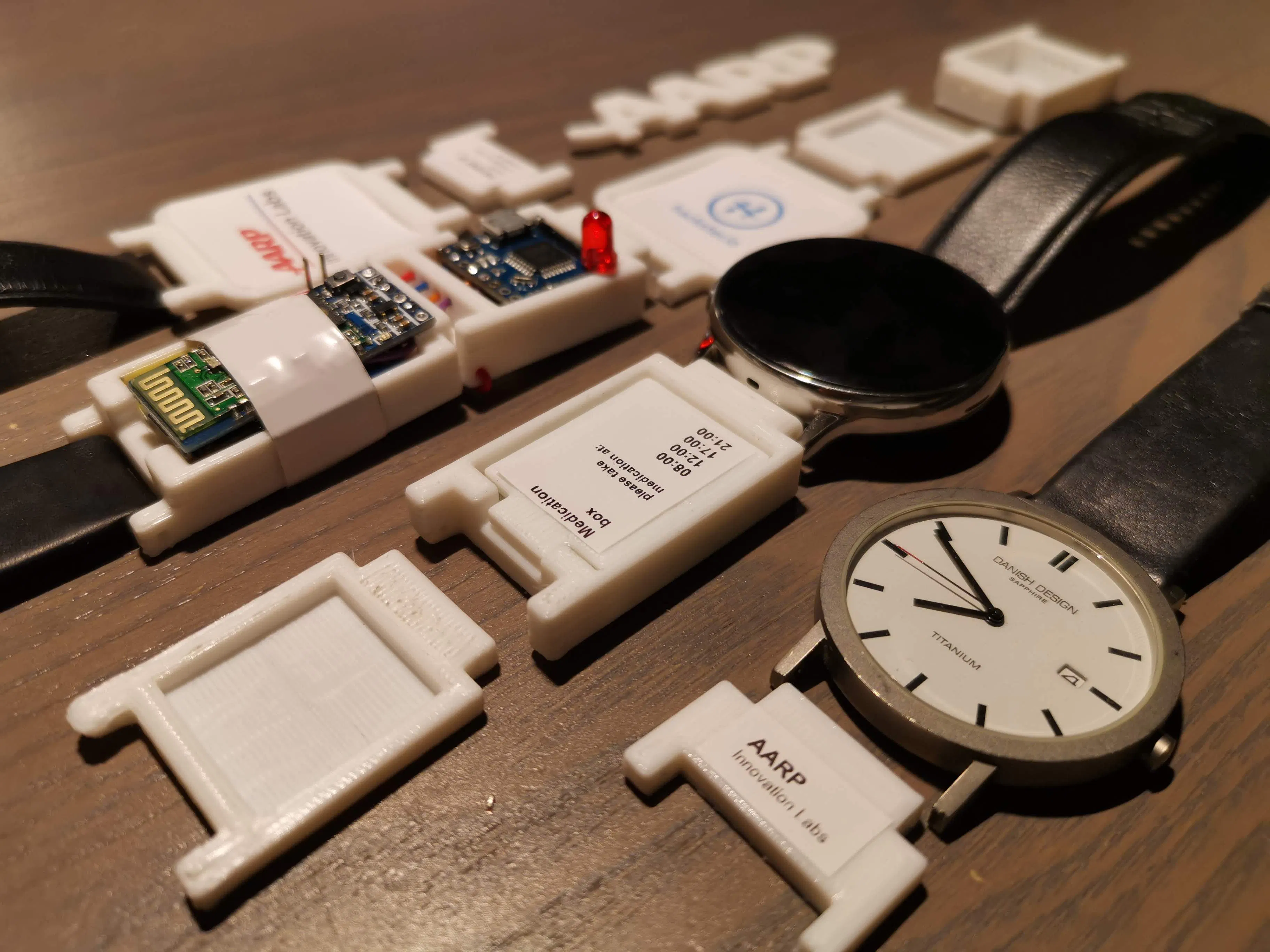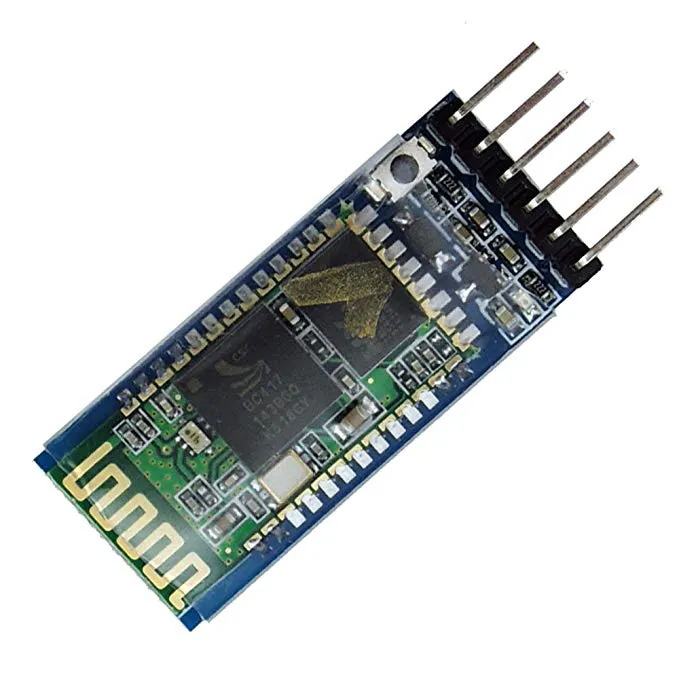# Modular Smart Watch/Bracelet

This watch has a modular design that combines digital and analog tools including fall detection, alarms, medication storage and photo frames

IntermediateShowcase (no instructions)3 days1,267## Things used in this project

### Hardware components

 Piksey Pico
×1
 MPU6050
×1HC-05 Bluetooth Module
×1

### Software apps and online servicesAndroid StudioArduino IDE

### Hand tools and fabrication machinesSoldering iron (generic)3D Printer (generic)

## Code

### Arduino code

Arduino
- Calibration data can be different on your hardware
- Check if your GPIO pin numbers are correct on your system
```#include<SPI.h>
#include<Wire.h>

int buttonPin = 4;
int count = 0;
int countSerialAvailable = 0;

int buttonState = 0;
int alarmState  = 0;
int alarmLEDpin = 14; // LED on the arduino

//variables for the MPU6050
int16_t AcX,AcY,AcZ,Tmp,GyX,GyY,GyZ, tempC;
float ax=0, ay=0, az=0, gx=0, gy=0, gz=0;

//int data[STORE_SIZE]; //array for saving  past data
//byte currentIndex=0; //stores current data array index (0-255)
boolean fall = false; //stores if a fall has occurred
boolean trigger1=false; //stores if first trigger (lower threshold) has occurred
boolean trigger2=false; //stores if second trigger (upper threshold) has occurred
boolean trigger3=false; //stores if third trigger (orientation change) has occurred

byte trigger1count=0; //stores the counts past since trigger 1 was set true
byte trigger2count=0; //stores the counts past since trigger 2 was set true
byte trigger3count=0; //stores the counts past since trigger 3 was set true
int angleChange=0;

void setup() {
Serial.begin(9600); // initialization 9600

// initialize the Alarm LED pin as an output:
pinMode(alarmLEDpin,OUTPUT);
digitalWrite(alarmLEDpin, LOW);
// initialize the pushbutton pin as an input:
pinMode(buttonPin, INPUT);

Serial.println("START");
}

void loop()
{

//2050, 77, 1947 are values for calibration of accelerometer
// values may be different for you
ax = (AcX-2050)/16384.00;
ay = (AcY-77)/16384.00;
az = (AcZ-1947)/16384.00;

//270, 351, 136 for gyroscope
gx = (GyX+270)/131.07;
gy = (GyY-351)/131.07;
gz = (GyZ+136)/131.07;

tempC = Tmp/340.00+36.53;

if(tempC >= 35)
{
Serial.println("HIGH TEMPERATURE DETECTED of ");
Serial.println(tempC);
Serial.println("C");

digitalWrite(alarmLEDpin, HIGH);
delay(5000);
digitalWrite(alarmLEDpin, LOW);
}

// calculating Amplitute vactor for 3 axis
float Raw_AM = pow(pow(ax,2)+pow(ay,2)+pow(az,2),0.5);
int AM = Raw_AM * 10;  // as values are within 0 to 1, I multiplied
// it by for using if else conditions

if (trigger3==true){
trigger3count++;
if (trigger3count>=10){
angleChange = pow(pow(gx,2)+pow(gy,2)+pow(gz,2),0.5);
//delay(10);
Serial.println(angleChange);
if ((angleChange>=0) && (angleChange<=10)){ //if orientation changes remains between 0-10 degrees
fall=true; trigger3=false; trigger3count=0;
Serial.println(angleChange);
}
else{ //user regained normal orientation
trigger3=false; trigger3count=0;
Serial.println("TRIGGER 3 DEACTIVATED");
}
}
}
if (fall==true){ //in event of a fall detection
Serial.println("FALL DETECTED");
digitalWrite(alarmLEDpin, HIGH);
delay(10000);
digitalWrite(alarmLEDpin, LOW);
fall=false;
// exit(1);
}
if (trigger2count>=6){ //allow 0.5s for orientation change
trigger2=false; trigger2count=0;
Serial.println("TRIGGER 2 DECACTIVATED");
}
if (trigger1count>=6){ //allow 0.5s for AM to break upper threshold
trigger1=false; trigger1count=0;
Serial.println("TRIGGER 1 DECACTIVATED");
}
if (trigger2==true){
trigger2count++;
//angleChange=acos(((double)x*(double)bx+(double)y*(double)by+(double)z*(double)bz)/(double)AM/(double)BM);
angleChange = pow(pow(gx,2)+pow(gy,2)+pow(gz,2),0.5); Serial.println(angleChange);
if (angleChange>=30 && angleChange<=400){ //if orientation changes by between 80-100 degrees
trigger3=true; trigger2=false; trigger2count=0;
Serial.println(angleChange);
Serial.println("TRIGGER 3 ACTIVATED");
}
}
if (trigger1==true){
trigger1count++;
if (AM>=12){ //if AM breaks upper threshold (3g)
trigger2=true;
Serial.println("TRIGGER 2 ACTIVATED");
trigger1=false; trigger1count=0;
}
}
if (AM<=2 && trigger2==false){ //if AM breaks lower threshold (0.4g)
trigger1=true;
Serial.println("TRIGGER 1 ACTIVATED");
}

if(buttonState != 0)
{
alarmState = 1;
Serial.print("BUTTON PRESSED");
}
else
{
if(count == 1)
Serial.println(".    ");
if(count == 2)
Serial.println(" .   ");
if(count == 3)
Serial.println("  .  ");
if(count == 4)
Serial.println("   . ");
if(count == 5)
Serial.println("    .");
if(count == 6)
Serial.println("   . ");
if(count == 7)
Serial.println("  .  ");
if(count >= 8){
Serial.println(" .   ");
count = 0;
}
}
if(alarmState == 1)
{
digitalWrite(alarmLEDpin, HIGH);
delay(2000);
alarmState = 0;
}
else
{
digitalWrite(alarmLEDpin, LOW);
}

//It appears that delay is needed in order not to clog the port
delay(100);
}

{
Wire.write(0x3B);  // starting with register 0x3B (ACCEL_XOUT_H)
Wire.endTransmission(false);
Wire.requestFrom(MPU_addr,14,true);  // request a total of 14 registers
}
```

### Android source code

Java
The source code for the Android application.
Android Studio is required to open the application (made in debug, API 27, Android 8.1, Gradle version 4.4, Android plugin 3.1.3)
`No preview (download only).`

### Android APK

Java
Android APK file.
Compatible with Android 8.1 or above.
`No preview (download only).`

## Credits

### Marcel Kruse

5 projects • 23 followers
Software & Hardware tester at an Embedded Systems company. Studied Electrical Engineering. Follow me on twitter: twitter.com/marcelkruse